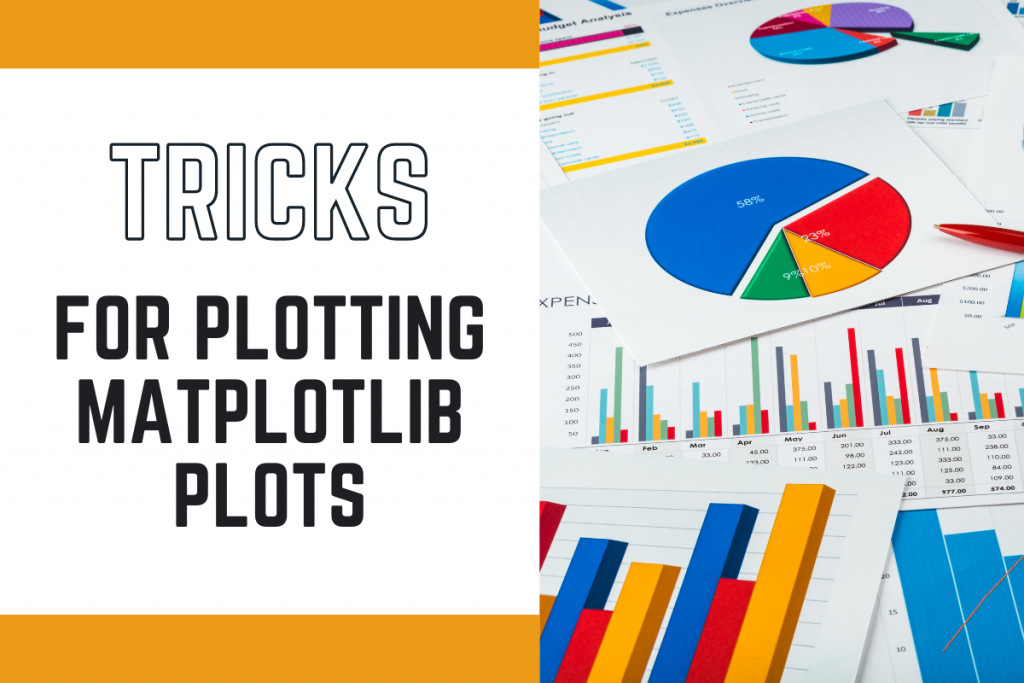# 3 Matplotlib Plotting Tips to Make Plotting EffectiveLet’s learn some matplotlib plotting tips today that will not only improve your plots visually but also help you make things easier. I am pretty sure you already know the popular plotting module known as matplotlib. But did you know there is so much more to explore in the module?

Today, we will be learning some unique unknown functions that exist in the module which can make your plot a lot better.

In case you are still unfamiliar with matplotlib library make sure you have a read to the following tutorials.

## Trick 1: Changing the size of the plot

Changing the size of a graph can help in better visualization of the plot. The same can be done with the help of the `figure` function and mentioning the width and height of the figure as `figsize`. The height and width are passed in inches.

Let’s look at the same through the code mentioned below.

```import matplotlib.pyplot as plt

x = [i for i in range(-10,10)]
y = [2*i*i*i + 4*i for i in x]

plt.title("Normal Sized Plot")
plt.plot(x,y)
plt.show()

plt.figure(figsize=(3,3))
plt.plot(x,y)
plt.title("Smaller version of the plot")
plt.show()
```

Both the plots are displayed below.

Annotations are comments added to a plot at the data points for making the graph more understandable and easy to analyze. Annotations can be added in two different ways: `text` and `annotate` function.

The implementation of both the methods are displayed below.

### 2.1: Annotations to Matplotlib Plotting using the `text` function

The code to add annotations using the text function is displayed below.

```x = [i for i in range(-2,2)]
y = [2*i*i + 4*i for i in x]
plt.title("Annotations using the text function")
plt.plot(x,y)

for i,j in zip(x,y):
# x_cordinate, y_cordinate, text, other properties
plt.text(i,j,"("+str(i)+","+str(j)+")",color="red")

plt.show()
```

### 2.2: Using the `annotate` function

Now have a look on how to add annotations using the annotate function with the help of the code below.

```x = [i for i in range(-2,2)]
y = [2*i*i + 4*i for i in x]
plt.title("Annotations using the annotate function")
plt.plot(x,y)

for i,j in zip(x,y):
t=(i,j)
# text_to_be_added, x and y cordinate in the form of tuple, other properties
plt.annotate("("+str(i)+","+str(j)+")",xy=t,color="red")

plt.show()
```

## Trick 3: Adding Watermarks to the plot

Watermarks are not commonly used in matplotlib, but they can be added if the user wants to own the plots.

You can have two types of watermarks:

1. Text-based Watermark
2. Image-based Watermark

To add watermarks to the plot we will create a subplot object which returns two figure objects. We can directly plot and add text and image watermarks on the plot using the `text` function and the `figimage` function.

### 3.1 Text-Based Watermark

The text function would need the x and y coordinates along with the text one needs on the plot with the necessary properties on the text. The code and output of the same are shown below.

```fig, ax = plt.subplots()
x = [i for i in range(-2,2)]
y = [2*i*i + 4*i for i in x]
ax.plot(x,y)

plt.show()
```

### 3.2 Image-Based Watermark

To add the image watermark we need to import the right module from the matplotlib library and then read the image using the `imread` function. We will then add the image using the `figimage` function on the second object of the subplot. The code and output are displayed below.

```import matplotlib.image as image

fig, ax = plt.subplots()

x = [i for i in range(-2,2)]
y = [2*i*i + 4*i for i in x]
ax.plot(x,y)

fig.figimage(img, 100, 100,alpha=0.5)

plt.show()
```